Try NerdPal! Our new app on iOS and Android

# Find the integral $\int\frac{4x^2-14x-6}{x^3-2x^2-3x}dx$

## Related Videos

Go!
Go!
1
2
3
4
5
6
7
8
9
0
a
b
c
d
f
g
m
n
u
v
w
x
y
z
.
(◻)
+
-
×
◻/◻
/
÷
2

e
π
ln
log
log
lim
d/dx
Dx
|◻|
θ
=
>
<
>=
<=
sin
cos
tan
cot
sec
csc

asin
acos
atan
acot
asec
acsc

sinh
cosh
tanh
coth
sech
csch

asinh
acosh
atanh
acoth
asech
acsch

### Videos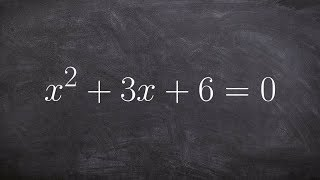### Algebra 2 - Learn how solve a quadratic by completing the square with fractions, x^2 + 3x + 6 = 0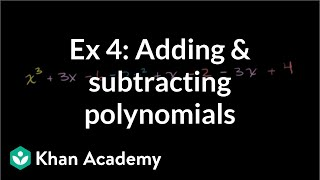### Example 4: Adding and subtracting polynomials | Algebra I | Khan Academy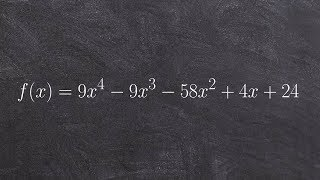### How to Use the Rational Zero Test to Help Find the Real Zeros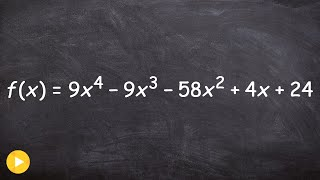### How to use the rational zero test to determine all possible rational zeros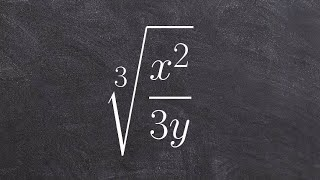### Algebra 2 - How to divide the cube root of a rational expression by rationalizing the denominator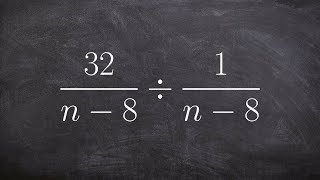SnapXam A2

### beta Got another answer? Verify it!

Go!
1
2
3
4
5
6
7
8
9
0
a
b
c
d
f
g
m
n
u
v
w
x
y
z
.
(◻)
+
-
×
◻/◻
/
÷
2

e
π
ln
log
log
lim
d/dx
Dx
|◻|
θ
=
>
<
>=
<=
sin
cos
tan
cot
sec
csc

asin
acos
atan
acot
asec
acsc

sinh
cosh
tanh
coth
sech
csch

asinh
acosh
atanh
acoth
asech
acsch

$\int\frac{4x^2-14x-6}{x^3-2x^2-3x}dx$

### Main topic:

Integrals by Partial Fraction expansion

~ 0.18 s